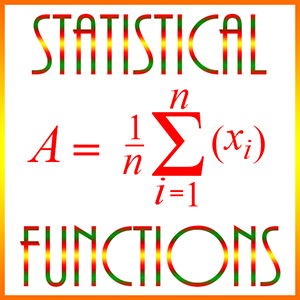# Statistical FunctionsStatistical functions normally refer to the (arithmetic) mean or the average, the median, the mode, the range of value in a set, the variance, and the standard deviation.

On this page we give 3 examples of the mean and the median.

Example 1)

If 8 boys in a gym class are 5 1/4 feet tall, and 4 boys are 6 feet tall, what is the average height of these boys?

A. 5 3/4 feet
B. 5 1/2 feet
C. 5 3/8 feet
D. 5 7/8 feet
E. 5 3/5 feet

Explanation: The average is the mean, and the mean is

((8 × 5 1/4 ) + ( 4 × 6))/12 = (42 + 24)/12 = 66/12 = 5 1/2

Example 2)

If y is the mean and x the median of the numbers, m+4, m-5, m-6, m+2, m+5, then what is x – y?

A. 0
B. 1
C. 2
D. 2m
E. 2m + 2

Explanation: First, we put the numbers in ascending order.

m-6, m-5, m+2, m+4, m+5

Then the median is the middle value of this list: x = m+2.

The mean is the average of these 5 numbers: y = (m-6 + m-5 + m+2 + m+4 + m+5)/5 = (5m – 11 + 11)/5 = 5m/5 = m.

So x – y = m + 2 – m = 2.

Notice that since we’re looking for x – y then the values for m subtract out, and we’re left with a literal number, 2. If instead we added x and y, we would get

x + y = m + 2 + m = 2m + 2, which is answer E.

Example 3)

From 20 summer lawn mowing jobs Teri averaged $18 per job. If she averaged only$15 on 8 of the jobs, what did she average on the other 12?

A. $22 B.$20
C. $21 D.$24
E. \$19

Explanation: Teri received total pay on the 20 jobs of 20 × 18 = 360. She got 15 × 8 = 120 on 8 of the jobs, so her average on the other 12 was

(360 – 120)/12 = 240/12 = 20

So B is the correct answer.